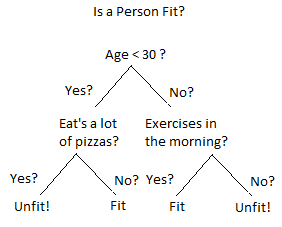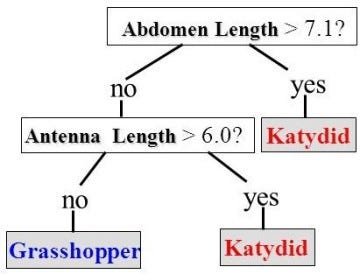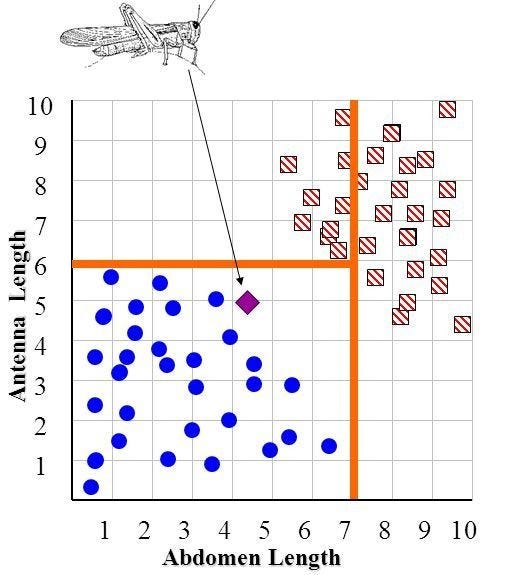# Decision Tree Classification

An introduction to Decision Tree Classifier

A Decision Tree is a simple representation for classifying examples. It is a Supervised Machine Learning where the data is continuously split according to a certain parameter.

Decision Tree consists of :

1. Nodes : Test for the value of a certain attribute.
2. Edges/ Branch : Correspond to the outcome of a test and connect to the next node or leaf.
3. Leaf nodes : Terminal nodes that predict the outcome (represent class labels or class distribution).Check if a person is Fit or Unfit using Decision Tree

To understand the concept of Decision Tree consider the above example. Let’s say you want to predict whether a person is fit or unfit, given their information like age, eating habits, physical activity, etc. The decision nodes are the questions like ‘What’s the age?’, ‘Does he exercise?’, ‘Does he eat a lot of pizzas’? And the leaves represent outcomes like either ‘fit’, or ‘unfit’.

## There are two main types of Decision Trees:

1. Classification Trees.
2. Regression Trees.

### 1. Classification trees (Yes/No types) :

What we’ve seen above is an example of classification tree, where the outcome was a variable like ‘fit’ or ‘unfit’. Here the decision variable is Categorical/ discrete.

Such a tree is built through a process known as binary recursive partitioning. This is an iterative process of splitting the data into partitions, and then splitting it up further on each of the branches.

### 2. Regression trees (Continuous data types) :

Decision trees where the target variable can take continuous values (typically real numbers) are called regression trees. (e.g. the price of a house, or a patient’s length of stay in a hospital)

### Creation of Decision Tree :

In this method a set of training examples is broken down into smaller and smaller subsets while at the same time an associated decision tree get incrementally developed. At the end of the learning process, a decision tree covering the training set is returned.

The key idea is to use a decision tree to partition the data space into cluster (or dense) regions and empty (or sparse) regions. In Decision Tree Classification a new example is classified by submitting it to a series of tests that determine the class label of the example. These tests are organized in a hierarchical structure called a decision tree. Decision Trees follow Divide-and-Conquer Algorithm.

### Divide and Conquer

Decision trees are built using a heuristic called recursive partitioning. This approach is also commonly known as divide and conquer because it splits the data into subsets, which are then split repeatedly into even smaller subsets, and so on and so forth until the process stops when the algorithm determines the data within the subsets are sufficiently homogenous, or another stopping criterion has been met.

### Basic Divide-and-Conquer Algorithm :

1. Select a test for root node. Create branch for each possible outcome of the test.
2. Split instances into subsets. One for each branch extending from the node.
3. Repeat recursively for each branch, using only instances that reach the branch.
4. Stop recursion for a branch if all its instances have the same class.

### Decision Tree Classifier

• Using the decision algorithm, we start at the tree root and split the data on the feature that results in the largest information gain (IG) (reduction in uncertainty towards the final decision).
• In an iterative process, we can then repeat this splitting procedure at each child node until the leaves are pure. This means that the samples at each leaf node all belong to the same class.
• In practice, we may set a limit on the depth of the tree to prevent overfitting. We compromise on purity here somewhat as the final leaves may still have some impurity.Decision Tree for Grasshopper and Katydid problemClassifying whether an insect is a Grasshopper or a Katydid based on Antenna Length and Abdomen Length.

### Advantages of Classification with Decision Trees:

1. Inexpensive to construct.
2. Extremely fast at classifying unknown records.
3. Easy to interpret for small-sized trees
4. Accuracy comparable to other classification techniques for many simple data sets.
5. Excludes unimportant features.

### Disadvantages of Classification with Decision Trees:

1. Easy to overfit.
2. Decision Boundary restricted to being parallel to attribute axes.
3. Decision tree models are often biased toward splits on features having a large number of levels.
4. Small changes in the training data can result in large changes to decision logic.
5. Large trees can be difficult to interpret and the decisions they make may seem counter intuitive.

### Applications of Decision trees in real life :

1. Biomedical Engineering (decision trees for identifying features to be used in implantable devices).
2. Financial analysis (Customer Satisfaction with a product or service).
3. Astronomy (classify galaxies).
4. System Control.
5. Manufacturing and Production (Quality control, Semiconductor manufacturing, etc).
6. Medicines (diagnosis, cardiology, psychiatry).
7. Physics (Particle detection).